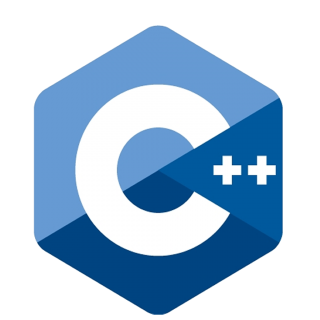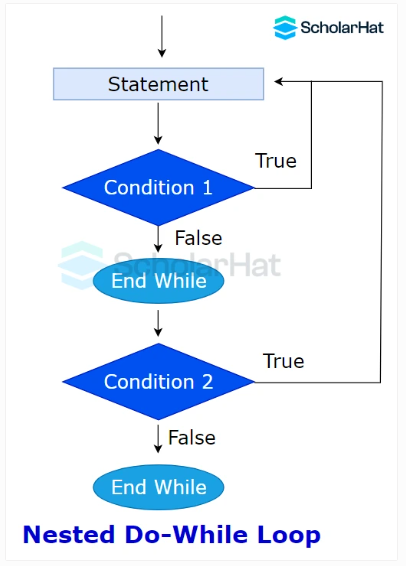# Understanding Do...While Loop in C++

24 Nov 2023
Beginner
112 ViewsLearn via Video Course & by Doing Hands-on Labs

## Do...While Loop in C++: An Overview

Now, it's time to look at the third and the last loop in our C++ programming tutorial i.e. the`do...while` loop. After understanding the for loop and while loop in detail, it will be effortless for you to understand the `do...while` loop in every aspect.

## Do...While Loop in C++ It is an exit-controlled loop. It prints the output at least once before checking the condition. Afterwards, the condition is checked and the execution of the loop begins.#### Syntax

``````do{
//code to be executed
}while(testCondition);
``````
• The body of the loop executes before checking the condition.
• If the `testCondition` is `true`, the loop body executes again.
• Again the `testCondition` is evaluated.
• The process repeats until the `testCondition` becomes `false`.

#### Example to demonstrate do...while loop in C++

``````#include <iostream>
using namespace std;
int main() {
int i = 0;
do {
cout << i + 1 << endl;
i++;
} while (i < 10);

return 0;
}
``````
• The above code prints numbers from 1 to 10 using the `do...while` loop in C++.
• It prints 1 before checking if `i<10`.
• It checks the condition `i<10` and executes until `i` becomes 10

#### Output

``````1
2
3
4
5
6
7
8
9
10
``````

### Nested Do...While Loop in C++

It is a `do...while` loop inside any `do...while` loop.#### Example to demonstrate Nested do...while loop in C++

``` ```#include <iostream>
using namespace std;
int main() {
int i = 1;

do {
int j = 1;
do {
cout << i << " * " << j << " = " << i * j << endl;
j++;
} while (j <= 10);
i++;
} while (i <= 2);

return 0;
}
``````
• The above code creates and outputs multiplication tables of 1 and 2 from 1 to 10 using a `nested` loop structure.
• It iterates over the values of `i` (1 and 2) and `j` (1 to 10), outputting the result of `i * j` after each iteration using two `nested do...while` loops.

#### Output

``````1 * 1 = 1
1 * 2 = 2
1 * 3 = 3
1 * 4 = 4
1 * 5 = 5
1 * 6 = 6
1 * 7 = 7
1 * 8 = 8
1 * 9 = 9
1 * 10 = 10
2 * 1 = 2
2 * 2 = 4
2 * 3 = 6
2 * 4 = 8
2 * 5 = 10
2 * 6 = 12
2 * 7 = 14
2 * 8 = 16
2 * 9 = 18
2 * 10 = 20
``````

### Infinite do...while loop in C++

It gets created when the `testCondition` remains `true`.

#### Syntax

``````do
{
// body of the loop
}while(true);
``````

#### Example to demonstrate infinite do...while loop in C++

``` ```#include <iostream>
using namespace std;
int main() {
do {
cout << "This is an infinite do-while loop." << endl;
} while (true);

return 0; // This return statement may never be reached.
}
``````

#### Output

``````This is an infinite do-while loop.
This is an infinite do-while loop.
This is an infinite do-while loop.
...
``````
##### Summary
We have completed all three loops in C++ programming. Loops in C++ have a broad range of applications, from loop-driven algorithms to iterative problem-solving. As demonstrated, the syntax for using these loops is relatively straightforward, although their logic must be carefully explored to determine advantage and ease of use. For more understanding, you can consider our C++ Certification program.
Share Article
Similar Articles